# Musimathics Errata

### Last Updated: 05/04/19

NOTE: The MIT Press released a second printing of Musimathics Volume 1 mid-September, 2008 that fixed all errata in the first printing of Volume 1 as of 7/15/2008. I have started a new table for errata that have appeared since the Second Printing. of V1, below. Though there have been additional printings since that time, there have been no further corrections to the printed text. So if you have a more current printing, you should still consult the Second Printing errata.

#### Determining the print version you own

Errata for Musimathics Volume 1 are different depending on which printing you have. Look at the bottom of the Copyright page. If the bottom of the Copyright page reads:

“10 9 8 7 6 5 4 3 2 1”, then you have the

10 9 8 7 6 5 4 3 2”, then you have the Second Printing.

The third, forth, etc. printing simply lop off the final digit. For any higher printing you should still consult errata for the Second Printing.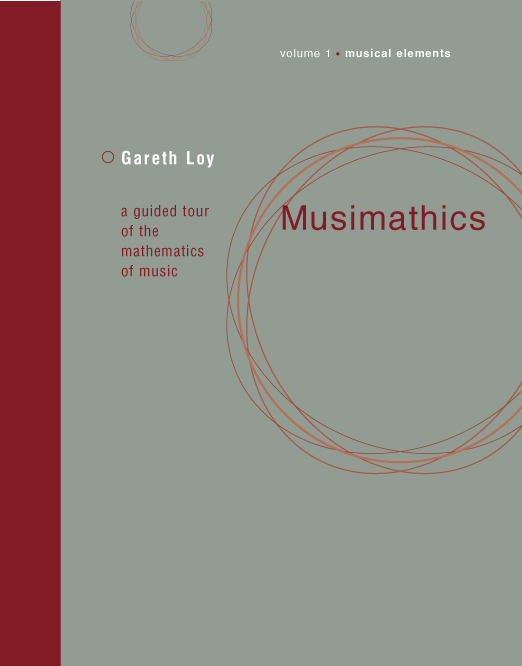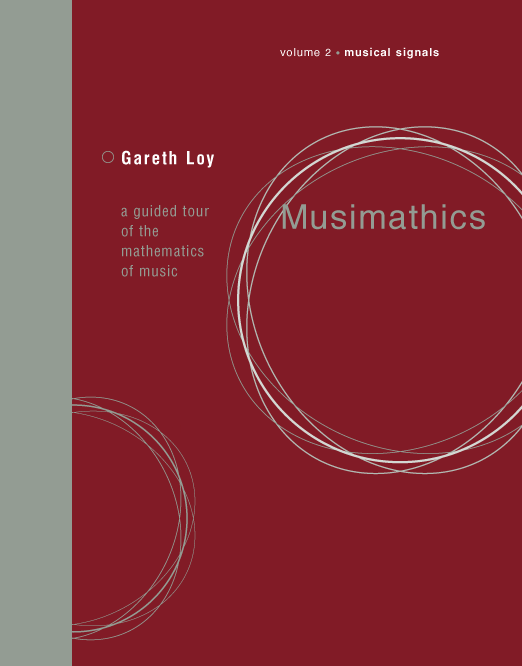Volume 1 – Musical Elements

Volume 2 – Musical Signals

Dr. Gareth Loy

# Musimathics Volume 1 Errata for Second and Subsequent Printings

Note: The second printing of Musimathics V1 corrected all known errata from the first printing. This table shows only the errata found in the second and subsequent printings.

#### Correction

p. 5

Correction

The sentence leading from the bottom of page 4 to page 5 is incorrect. It reads, “The spring begins to go slack as the mass rises, and when the mass reaches the equilibrium point, the spring no longer lifts the mass upward.”

It should read instead, “The spring begins to go slack as the mass rises, and when the mass reaches the equilibrium point, the force due to gravity is greater than that of the spring.”

p. 17
§ 2.4.2
1st
and Fig. 2.3

Solmisation syllable “ti” vs. “si”

Solmization syllables in English countries use “ti” for the 7th degree while many other countries use “si”. The text and figure should have a footnote explaining this regional difference. (Strictly speaking, not an erratum.)

p. 19
Table 2.1

Augmented & Diminished intervals

The number of semitones should not be shown for Augmented & Diminished intervals. Yes, the augmented fourth and diminished fifth each have 6 semitones, but because these terms can be applied to other intervals as well, this is confusing. It would be better either not to indicate the number of semitones for Augmented and Diminished, or perhaps to give “+1” and “–1” for them to indicate that what they really do is to add or subtract a semitone to the named interval, respectively.

p. 20

Correction

Text reads,

The Lochrian mode is purely a theoretical mode, considered unusable by conventional music theory because of the tritone that exists between its final (7) and its fourth degree.”

Text should read,

The Lochrian mode is purely a theoretical mode, considered unusable by conventional music theory because of the tritone that exists between its final (I) and its fifth degree (V).”

p.22
Fig. 2.10.b

Typo in figure

Pitch labeled “B” should be “Bb” (“B-flat”).

p. 23
Fig. 2.12b

Alignment in figure

The bar on F Major has the flat in the position of A instead of B. The flat symbol should be nudged up a bit.
p. 24
Fig. 2.14

Additional information

The printed spelling of the pentatonic scale shown in Fig. 2.14 is just one of several modes (circular permutations) of the scale's intervallic sequence, {2, 3, 2, 2, 3} discussed on page 23; there is no “official” pentatonic mode, and one is as good as another so long as the interval order is preserved. If I had it to do over again, I might have chosen the mode {2, 2, 3, 2, 3}, as it outlines a major triad in root position.

p. 24
Fig. 2.15

Typo

Pitch labeled “A” should be labeled “Ab” (“A-flat”).

p. 26
Table 2.3

Whole note rest is incorrect

The way I learned it in school, the whole-note rest “hat” is “off”, and the half-note rest “hat” is “on”. Evidently, I need to go back to school on this one. The whole-note rest should look like this:p. 31
Fig. 2.21

Typo

Top-left text reads “Fundamental 1”, should read just “Fundamental”.

p. 34

Fig. 2.24

Instrument plot from Grey 1975 is probably an Eb clarinet tone, not a stringed instrument tone.

p. 42
just before
§ 3.2.2

Arguments to function f are reversed.

The function is defined on page 41 bottom as f (k, v),
but the examples of use on page 42 reverse the arguments: f (v, k).
For example, the text reads, “A0 = f (0, 9)” but should read “A0 = f (9, 0)”.
Here are the function arguments as shown in the text:
Here are the corrected function arguments:
p. 43

Correction

Text reads: “We can use equation (3.1) to add and subtract intervals. If k = 2 in that equation, then frequency fk will be two octaves above frequency fR.”

Text should read: “We can use equation (3.3) to add and subtract intervals. If v = 2 in that equation, then frequency fk,v will be two octaves above frequency fR.”

p. 46
first full ¶

Correction

Text reads,

So the tempered fifth is almost 2 cents flat of a perfect fifth.”

Text should read,

So the tempered fifth is almost 2 cents flat of a just fifth.”

p. 59
Fig. 3.11

Correction

Last interval (bottom left of figure) labelled “Maj. 6Th 8/5” should be “Min. 6th 8/5”.

p. 78
Table 3.9

Correction and clarification

Text on p. 78 describing Table 3.9, “Partch's 43-Tone Scale” (which appears on p. 77) incorrectly describes the highlighting and outlining shown in the table. The interval groups are correct, but the description of their highlighting and outlining is not.

For example, “Intervals of approach” (#2 - #10 and #35 - #43) are said to be “shown with a light outline”, but are not outlined in the table. Likewise, the “Intervals of suspense” (#19 - #26) are incorrectly described as having light shading, however the first and last row in the region is white, while the rows in between are shaded the same as all shaded rows.

p. 80
Fig. 3.25

Typo

The 9th degree of the 22-sruti scale is mislabeled as an 81/84 ratio; this should actually read 81/64. The ratio is given correctly in Table 3.10 on the previous page.

p. 80

Correction

End of first full paragraph under Figure 3.26, the text says,

the size of the murchana interval corresponds to the Pythagorean comma”,

however the text should read,

the size of the murchana interval corresponds to the Syntonic comma”.

p. 118
first ¶ after § 4.22
2nd sentence

Incorrect word

Text reads: “Intensity I is the energy E per unit of time t that is flowing across a surface of unit area a

Delete “unit”, since intensity is the power in the area a, whatever its size.

Text should read: “Intensity I is the energy E per unit of time t that is flowing across a surface of area a

p. 118
Eq. 4.34

Incorrect term in equation

The denominator should be a, not a2. We divide power by the area, not the square of the area. The equation could then be more simply expressed:

I = E / t / a.

p. 118
text line just below
eq. 4.34

Incorrect term in equation

Inline equation reads: “I = P / a2”, but should read “I = P / a” to correct the derivation from the revised Eq. 4.34.

p. 119
first ¶

Improvement

Text reads:

Let P be the power of a wave at distance r1 propagating along direction V. This means that an amount of energy P is flowing through surface a1 each second. If no energy is lost, then the same power will flow through a2 each second as well, and P/a1 = P/a2. Since the areas of surfaces a1 and a2 are proportional to the squares of their distances from the source S, the intensity I varies inversely as the square of the distance to the source...”

Substitute:

Let P be the power of a wave at distance r1 propagating along direction V. This means that an amount of energy P is flowing through surface a1 each second. If no energy is lost, then the same power will flow through a2 each second as well, so total power P at a1 would be the same as at a2. However, since the areas of surfaces a1 and a2 are proportional to the squares of their distances from the source S, the intensity I varies inversely as the square of the distance to the source...”

p. 119
Eq. 4.37

Incorrectly identifies a unit for a unitless ratio

Eq. 4.37 expresses the ratio of (limit of hearing)/(threshold of hearing) in units of W/m2, but this ratio is dimensionless and so has no units. Think of it this way: since the limit of hearing and threshold of hearing are both expressed as W/m2, the ratio of these units cancel, resulting in a dimensionless ratio.

p. 123

Units for average pressure variation are incorrect

Mid-page, in the paragraph beginning “Using standard values...”, the expression at the end of that paragraph should be 2 ∙ 105 N/m2.
p. 123

Values for threshold and limit of hearing are incorrect

Second to last sentence gives incorrect values for the threshold of hearing and limit of hearing. The value for the threshold of hearing should be 20 micropascals, not “0.1 Pa”, and the value for the threshold of hearing should be 20 pascals, not “1 Pa”.

p. 125
2nd
from bottom

Incorrect term

Text reads:

We can distinguish linear distance, area distance, and volumetric distance.”

Change each word “distance” to “density”.

We can distinguish linear density, area density, and volumetric density.”

p. 127
2nd full ¶

Too much (redundant) information

Two consecutive sentences essentially repeat the same point with slightly different wording: “For a sound wave, amplitude is the difference between the wave's peak pressure and standard atmospheric pressure. Sound amplitude is usually measured as sound pressure level, which is the difference between the greatest pressure in a wave and standard atmospheric pressure.”

Brought to your attention by the Musimathics Department of Redundancy Department

p. 134 “rocket ship” example

Correction and clarification

In the first full paragraph, change the second sentence (“To get it to turn...”) to say, “If it is moving with linear velocity v, we would need to fire an engine on its side, pointing toward the circle's center, having force proportional to v2/r.”

p. 139 Figure 5.10

Extraneous “1” near origin

An extraneous character “1” appears just to the left of the axis origin.

p. 139

Incorrect formula

In the formula at the very bottom of the page, the first three “sin” terms should be removed. (The 4th is to be preserved.) The formula should read: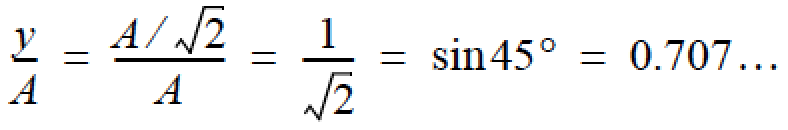p. 140
§ 5.4.1

Deprecated passage

Section 5.4.1 introduces definitions of time t (5.20) and frequency f (5.21) that are confusing, unnecessary, and unused in subsequent text. Please disregard ¶2 through ¶4 of that section. That is, ignore from the ¶ beginning, “It will often be more convenient...” to the ¶ ending, “we are measuring periodicity” (found just above the last ¶ of the page).

p. 143

Incorrect terms in equations

In the last full sentence of the page, v = d/t should be v = d/T and v = 2πA/t should be v = 2πA/T.

p. 144

Incorrect terms in equations

In the first sentence of the page, v = 2πA · (1/t) should be v = 2πA · (1/T), and f = 1/t should be f = 1/T.

p. 145

Incorrect terms in equation

In the first full paragraph, the text reads, “so in general l +l ≅ 0”.

The text should read: “so in general | l + | – | l | ≅ 0”.

In English the meaning is as follows: because sound waveforms are usually evenly balanced above and below ambient pressure, so in general, the magnitude of the highest peak level minus the magnitude of the lowest trough level are approximately equal to zero.

p. 147

Text is incorrect and insufficient

First sentence reads: “Decibels of sound pressure level (SPL) correspond to twice the equivalent decibels of sound intensity level (SIL).”

Please replace this with: “Because they expresses ratios of quantities having the same unit, the dB and bel scales are dimensionless measures. Thus, to have meaning, the thing being measured (for example, SPL or SIL) must be specified explicitly. Saying 3 dB by itself is meaningless; however saying 3 dB SIL indicates a doubling of intensity.”

Second sentence begins, “Where ...”; please replace with, “Note that...”.

p. 152

Typo (terms are switched)

First sentence on page reads in part:

... known as the hammer (incus), the anvil (malleus) ...”

but should read,

“ … known as the hammer (malleus), the anvil (incus) ...”

p. 161
Figure 6.3a

Inverted axis

The labels on the positive y axis of Figure 6.3a are inverted: “2” above, “4” below; but should be “4” above, “2” below.

p. 174 Figure 6.14 caption text

Citation for Roederer is incorrect

Text should read, “Adapted from Roederer 1973.”

p. 177
Fig. 6.16

Typo

Vertical axis says “phons”, but should say “phon”.

p. 181
Table 6.1

Incorrect bandwidth for Bark #13

The Critical Bandwidth for Bark #13 should be ~ 320 Hz, not 100 Hz.

p. 182
Eq. 6.9

Unclear equation

Equation 6.9 “Bark Number” should have another set of brackets for definiteness:

z ( f ) = 13 atan (0.00076 f ) + 3.5 atan ( ( f / 7500 )2 )

p. 203

Incorrect word

The penultimate paragraph reads “whether we hold pressure or velocity constant”.

It should read “whether we hold pressure or volume constant”.

p. 204

Disambiguation

Variable m is given two definitions on this page. To clarify their different usages, substitute M for m in Eq. 7.5 and surrounding text. Specifically, in the second full ¶, change the text to read “... gas is its mass M per unit of volume V”. Then in Eq. 7.5 “Mass Density” also change m to M:

ρ = M / V

Then the definition of m in Eq. 7.6 will not conflated with the “min Eq. 7.5.

Note, the definition of m in 7.6 is used again in section 7.4.5, page 206.

p. 205

Correction to text

At the end of ¶2, the text reads, “... between 10–7 N/atm and 10–5 N/atm.”

Replace with: “... between 10–6 N/atm and 10–5 N/atm.”

p. 181
Fig. 6.19

Incorrect Q for critical band #15

Critical Band #15 sticks out like a long pole in a tent. It should be ~ 6.44 (because 2900/450 = ~6.44).

p. 249
§ 8.4.2

Missing preposition

Fourth sentence from bottom of that section reads in part “... creating an infinite variety sinusoidal motions ...” but should read “... creating an infinite variety of sinusoidal motions ...”.

p. 259

Incorrect number in text

End of the penultimate paragraph, the text reads, “... to an increase in k by a factor of about 186.”

Please substitute, “... to an increase in k by a factor of about 108.”

p. 287
Fig. 9.3

A quibble

Fig. 9.3 does not show the original “Ut queant laxis...” chant melody. The original chant melody is as shown.*
*This assumes the output of Guido's Method given in the figure would reproduce the original melody exactly, which is exceedingly unlikely. Strictly speaking therefore, it is not an erratum not to show the original, however, because the original is historically important, I make an exception.

p. 301

Typo

In the inline table above §9.7.3 showing shifting f = 0.2615 by b = 10, there is a typo in the second-to-last line.

The text reads “26.15 ∙10 = 26 1.15” but should read “26.15 ∙10 = 26 1.5”.

p. 310

Typo

Last sentence at bottom of page should refer to formula 9.4 not 9.5.

Sentence should read: “So we can use the partitioning formula (9.4), as follows:”

p. 315

Typo

Missing curly-brace.

Text reads: “So we can write I ({4,6,7,10}) = (8,6,5,2}

Should be: “So we can write I ({4,6,7,10}) = {8,6,5,2}

p. 322

Improvement

Regarding the discussion of transposing at the bottom of the page, a transpose method has already been defined at the top of page 318 that we can use, rather than define a new one on page 322, and the code here will be simpler. The transpose method defined on page 322 takes two Integer arguments, and the code at the bottom of the page uses a For list to iterate an IntegerList. But using the IntegerList transpose method defined on page 318 makes the code at the bottom of page 322 much simpler, as follows.

IntegerList Cmaj = {C, D, E, F, G, A, B}; // define C major scale

transpose(Cmaj, 4 * 12); // let the transpose method loop for us

Print(Pitch(Cmaj));

And that means we can ignore/delete the transpose method defined on page 322.

p. 335

Textual inaccuracy

Second full ¶, the text states “… the distribution of the sum … is the convolution ….”, however this is true only if the random variables being summed are independent; it is not generally true. Please make the following substitution.

A fundamental insight of probability theory is that if a random variable x has distribution f ( x ) and an independent random variable y has distribution f ( y ), then the distribution of the sum of the two random variables f ( x+y ) is the convolution of f ( x ) and f ( y ). (Intuitively, two random variables x and y are independent if knowing the value of x does not change the probabilities of y.)”

p. 342

Misleading code indenting

Well, the randomMelody function works, but how it works is a little counterintuitive due to misleading code indenting toward the bottom of the function. If you're already a code wizard you probably didn't notice a problem, but if you are not, this could look confusing. Here's the deal: indenting is always merely cosmetic; its the logical structure of the code, not the indenting, that matters. Indenting can help clarify for the reader what the code is doing, and so here I let you down. Sorry. So here's the code from the final Else clause near the bottom of the function to its end as it appears in the book: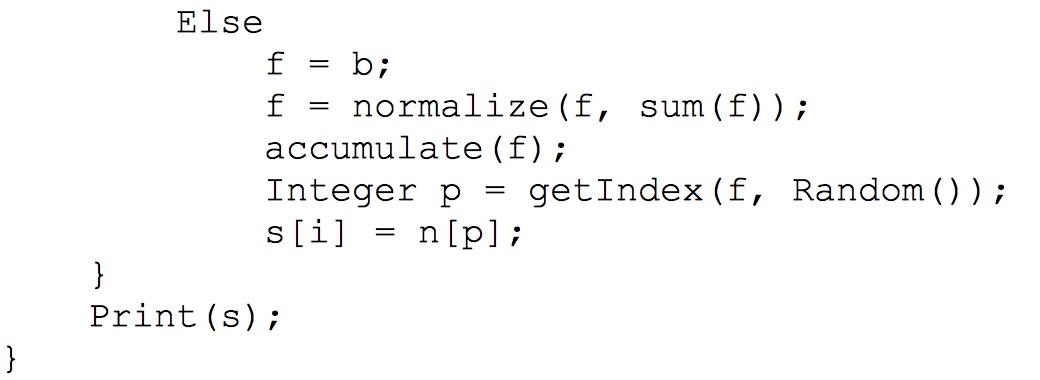Here is the way the code should have been indented in the book to clarify its structure: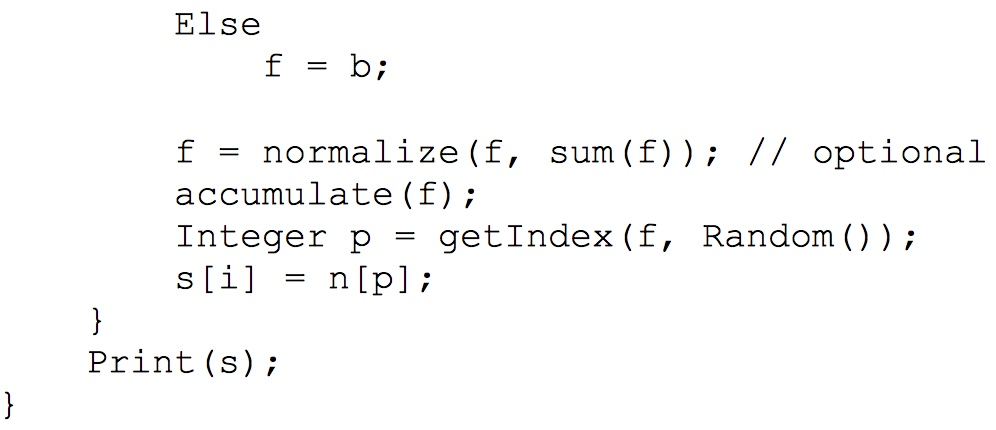This indenting confirms that, by default, an Else statement not followed by a compound statement (i.e., a code block contained in curly braces, { … }) must be followed by a single statement — in this case, the assignment of “f = b”. Thus, the last four lines of the enclosing For loop (from “f = normalize …” down to “s[i] = n[p]” is not a part of the final Else statement — these four lines are executed at the end of every iteration of the For loop.

Oh, and by the way, there's also a redundancy in the code. We could skip the line marked “// optional”. Can you figure out why?

p. 346
second line

Typo

corresponding surpisal” should be “corresponding surprisal”

p. 373, first sentence of the second bulleted paragraph

Typo

themeslves” should be “themselves”

p. 377
first ¶
after §9.22.2

Incorrect citation

Mid-paragraph, the text reads

In the network shown in figure 43b”,

but should read,

In the network shown in figure 9.42b”.

p. 398
fifth ¶
after §9.23.2

Incorrect formula

M0(PianoImprov) = (2 · A4) + (3 · B4) + (2 · G4)”

should read:

M0(PianoImprov) = (2 · C4) + (3 · E4) + (2 · G4)”

p. 401, last line

Typo

"truely" should be "truly"

p. 403

Misspelled word: “answsers”

Text should read: “EMI’s analysis database is essentially a compendium of answers to the question, What would Mozart have done in this situation?”

# Musimathics Volume 1 Errata – First Printing

Note: All of these errata were corrected in the second printing, and so this table is no longer updated. Refer to the table above for errata discovered after the second printing.

#### Correction

p. 5

Incorrect statement

The sentence leading from the bottom of page 4 to page 5 is incorrect. It reads, “The spring begins to go slack as the mass rises, and when the mass reaches the equilibrium point, the spring no longer lifts the mass upward.”

It should read instead, “The spring begins to go slack as the mass rises, and when the mass reaches the equilibrium point, the force due to gravity is greater than that of the spring.”

p. 14
last line

Sentence should read as corrected.

Real numbers include all integers and all possible fractional and irrational values.”

p. 20

Correction

Text reads,

The Lochrian mode is purely a theoretical mode, considered unusable by conventional music theory because of the tritone that exists between its final (7) and its fourth degree.”

Text should read,

The Lochrian mode is purely a theoretical mode, considered unusable by conventional music theory because of the tritone that exists between its final (I) and its fifth degree (V).”

p.22
Fig 2.10.b

Penultimate note in F major scale is E not E#.

Remove “#” after E.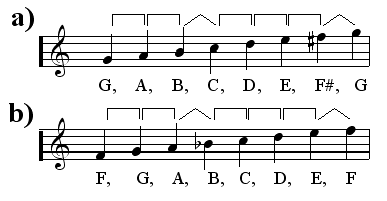p. 26
Table 2.3

Whole note rest is incorrect

The way I learned it in school, the whole-note rest “hat” is “off”, and the half-note rest “hat” is “on”. Evidently, I need to go back to school on this one. The whole-note rest should look like this:p.29
Fig 2.19

Plus signs in figure are incorrect

The plus signs in the list “f + 2f + 3f + ...” are mathematically meaningless. Fig. 2.27 better represents partials. A simple fix is to replace “+” with “,”, as shown below, representing the overtone frequencies as a series of integer multiples of a fundamental frequency. Alternately, to express the waveform that results from the combination of these sine components, let p(x) = sin(2 pi x t) where t is time, and then write p(f) + p(2f) + p(3f) + ....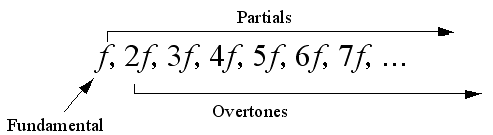p. 29
§ 2.8.1

Change heading

(Not really an erratum, but it needs a better title.)

Heading reads: “Partials, Fundamentals, and Overtones”

Change to read “Fundamental, Partials, Harmonics, and Overtones”

p. 42 just before Sec. 3.2.2

Arguments to function f are reversed

The function is defined on page 41 bottom as f(k,v), but the examples of use on page 42 reverse the arguments: f(v,k). For example, the text reads, “A0 = f(0,9)” but should read “A0 = f(9,0)”. Here are the function arguments as shown in the text:Here are the corrected function arguments:p. 43

Incorrect statement

Text reads: “We can use equation (3.1) to add and subtract intervals. If x = 2 in that equation, then frequency fx will be two octaves above frequency fR.”

Text should read: “We can use equation (3.3) to add and subtract intervals. If v = 2 in that equation, then frequency fk,v will be two octaves above frequency fR.”

p.43
Fig. 3.1

Plus signs are mathematically wrong

This is similar to the error on p. 29, Fig 2.19. The simplest fix is to remove the “+” signs. Alternately, the waveform can be expressed as a sum of sine components using the function p(x) as described above.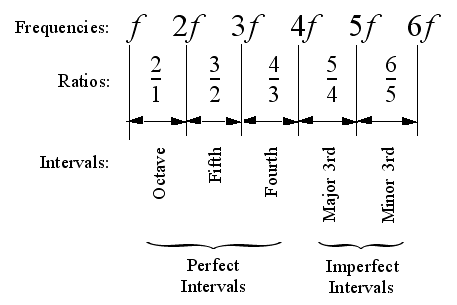p. 45 top Figures 3.2, 3.3,

also p. 49 at the bottom figure 3.5 at the bottom

The ratio for C is given as 1/2

The ratio for lowest pitch C should be 1/1, so that the octave above (2/1) would be correct.

p. 46 first full paragraph

Correction

Text reads,

So the tempered fifth is almost 2 cents flat of a perfect fifth.”

Text should read,

So the tempered fifth is almost 2 cents flat of a just fifth.”

p. 48 bottom, 7th sentence up from the bottom starting with "2.  The fifth is found by taking....."

Ratio is shown as 12:9

Ratio should be 9:6.

p. 104 Equation (4.12) and (4.13) Average Acceleration

Formulas (4.12) and (4.13) and their discussion are incorrect and misleading

Please replace the entire section “4.10 Acceleration” with this PDF file.

p. 111

Section 4.14.1 presents a wrong and a misleading derivation of kinetic energy. Equation (4.28) is missing a factor of 1/2

Please replace the entire section “4.14.1 Kinetic Energy” with this PDF file.

p. 111 last line

Misspelling

posses” should be spelled “possess”.

p. 119

Improvement

Text reads: “Let P be the power of a wave at distance r1 propagating along direction V. This means that an amount of energy P is flowing through surface a1 each second. If no energy is lost, then the same power will flow through a2 each second as well, and P/a1 = P/a2. Since the areas of surfaces a1 and a2 are proportional to the squares of their distances from the source S, the intensity I varies inversely as the square of the distance to the source...”

Substitute: “Let P be the power of a wave at distance r1 propagating along direction V. This means that an amount of energy P is flowing through surface a1 each second. If no energy is lost, then the same power will flow through a2 each second as well, so total power P at a1 would be the same as at a2. However, since the areas of surfaces a1 and a2 are proportional to the squares of their distances from the source S, the intensity I varies inversely as the square of the distance to the source...”

p. 119, Equation (4.36)

Incorrect equation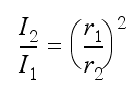Intensity of a sound does not increase with the square of the distance from a sound source but with its inverse. Corrected equation is shown here. Note terms r1 and r2 have been switched.

p. 132 Equation (5.8)

Angular Acceleration Equation (5.8) is incorrect and misleading, similar to problem on p. 104.

Equation (5.8) should read as corrected.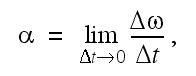p.134, bottom, in bold-italic text:

Improvement in wording to clarify concept.

Should read, “Circular motion is the result of a centripetal force applied at right angles to the instantaneous velocity.”

p. 139 Figure 5.10

Extraneous character

An extraneous character “1” appears just to the left of the axis origin. It should be removed.

p. 143, sec. 5.5

2nd para. 1st sentence: remove ref. to E_k. Also, E_k=mv^2 is missing a factor of 1/2

Sentence and equation should read as corrected.
p.144

Equation (5.27) and the sentence enclosing it are missing a factor of 1/2.

Sentence and equation should read as corrected.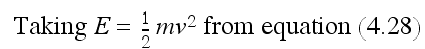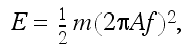p. 152

Typo (terms are switched)

First sentence on page reads in part:

... known as the hammer (incus), the anvil (malleus) ...”

but should read,

“ … known as the hammer (malleus), the anvil (incus) ...”

p. 174 Figure 6.14 caption text

Citation for Roederer is incorrect.

Text should read, “Adapted from Roederer 1973.”

p. 181 Table 6.1

Incorrect values for Bark #0 Lower Band Edge and Critical Bandwidth

Per [Zwicker 1961]: Bark 0 should be 0Hz for Lower Band Edge, 100Hz for Critical Bandwidth.

p. 181 Table 6.1

Incorrect bandwidth for Bark #13

The Critical Bandwidth for Bark #13 should be ~ 320 Hz, not 100 Hz.

p. 181 Fig. 6.19

Incorrect Q for band 15

Critical Band #15 sticks out like a long pole in a tent. It should be ~ 6.44 (because 2900/450 = ~6.44).

p.193 – 194
§ 6.13.5

Incorrect text

Text incorrectly implies that if a speaker has intensity I, then at twice the distance the intensity is sqrt(I), whereas in fact it will be I/4. This mistake affects each equation on this page: sqrt should be replaced by a factor 1/4, and squaring by a factor of 4. Please replace the entire section “6.13.5 Distance Cues” with this PDF file.

p. 203

Incorrect word

The penultimate paragraph it reads “whether we hold pressure or velocity constant”. It should read “whether we hold pressure or volume constant”.

p. 204 paragraph immediately below Eq. (7.5)

Incorrect text

Text states “To determine the speed of sound we must determine the mass density of air...” However, I just gave the mass density of air in the previous sentence! What we really need is the average molecular mass of air. “To determine the speed of sound, we must determine the average molecular mass of air...”

p. 210 sec. 7.8 2nd para. 4th sentence

Incorrect spelling

lightening” should be “lightning”

p. 226 Figure 7.24

The arrow labeled “Wavelength increases” should point up, not down.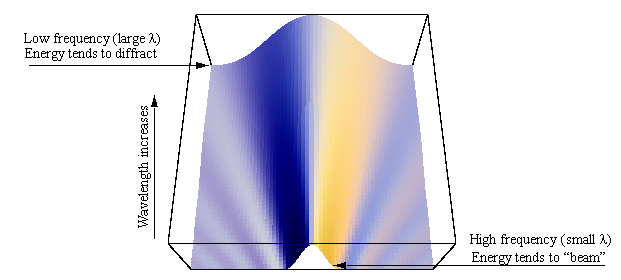p. 232 Equation 7.26

Incorrect formula

Formula for Doppler Shift in Two Dimensions is incorrect. The term u appearing inside the parentheses in the denominator should be outside the parentheses, as shown here.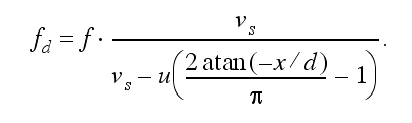I have created a Mathematica notebook that explains this equation better which is available here. A free player of Mathematica notebooks can be downloaded here. For a vector formulation of Doppler shift, see http://ccrma.stanford.edu/~jos/pasp/Doppler_Effect.html

p. 246 mid-page (5th paragraph)

Incorrect formula V = 7.5^ –4 m^3,

should be V = 7.5 x 10^ –4 m^3.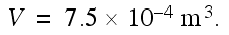p. 257
Fig. 8.13

Figure subheadings are incorrect. Should read as corrected.

a) Fundamental (first harmonic)

b) Second harmonic

c) Third harmonic

p. 260 sentence before run-in heading “Bar with Free Ends”

Sentence reads, “Plugging these values into (8.18) for n = 1,2,3,4,5 yields a fundamental and partials shown in the last column of table 8.3.”

Sentence should read, “Plugging these values into (8.18) yields a fundamental and partials shown in the last column of table 8.3.”

Delete “for n = 1,2,3,4,5”, there is no n in eq. 8.18.

p. 346, second line

Typo

"corresponding surpisal" should be "corresponding surprisal"

p. 373, first sentence of the second bulleted paragraph

Typo

"themeslves" should be "themselves"

p. 401, last line

Typo

"truely" should be "truly"

p. 359 Third sentence into section 9.17.5

It would be better to say that Voss & Clark used a Gaussian noise generator than a uniform noise generator.

Text reads:

To test this hypothesis, they synthesized melodies of three types using a computer: the first type made tone and rhythmic selections with a uniform...”

But should read:

To test this hypothesis, they synthesized melodies of three types using a computer: the first type made tone and rhythmic selections with a Gaussian...”

p. 396

Description of the Fusion Petri net structure is misleading

The text reads, “Only one of the two input places can trigger M1,” however this does not agree with the structure of the diagram to the right of that paragraph, nor with the definition of the Petri net firing rule given on page 391.

The text should read:

Both of the two input places are needed to trigger M1.”

p. 403

Misspelled word: “answsers”. Text should read:

EMI’s analysis database is essentially a compendium of answers to the question, What would Mozart have done in this situation?

p. 460

End Note 4 in “Chapter 5” last sentence states incorrectly, “Instead, circular motion is the vector sum of centripetal force and linear velocity.”

Corrected text should read:

Circular motion is the result of a centripetal force applied at right angles to the instantaneous velocity.”

# Musimathics Volume 2 Errata

(Volume 2 errata cover the hardbound and softbound editions. Some errata appear only in the softbound version.)

#### Correction

p. 23
first ¶ after §1.5.2

Clarification

The text names “the ones place”, “the tens place”, etc., without defining them. When referring to the representation of decimal numbers using place notation, the convention is to say “the ones place” for the rightmost digit, “the tens place” for the digit to its left, “the hundreds place”, etc., for subsequent digits to the left.

p. 30
sentence before fig. 1.24

Incorrect units

Text reads: “In this example, values of x in the range –1/4 to nearly 1/4 map to the reproduction level value of 0.”

Text should read: “In this example, values of x in the range –1/8 to nearly 1/8 map to the reproduction level value of 0.”

p. 39
section 1.11.1

Incorrect calculation

In the section titled “Storage Requirements of Digital Audio” I discuss how much space is needed to store a certain amount of audio. Part of the calculation is correct, up to the point where I say that we require about 0.1764 megabytes/s. However, then I claim that this amounts to about 340 megabytes for one hour. This is incorrect. At 0.1764 mb/s, this means about 10.584 mb/minute (multiplying by 60). Multiplying this by 60 again, we end up with about 635.04 mb/hour, the correct figure. (It seems that what I did to arrive at 340MB/hour is to multiply the 5.67 seconds per byte by 60!) Doh!

p. 65
eq. 2.31

Incorrect term

The last term in the expression reads: \frac{7^{7}}{7!} but should read: \frac{z^{7}}{7!} Please substitute the following.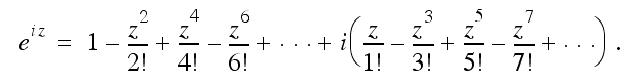p. 77
sentence just before §2.6.8

Textual improvement

Text reads: “We can see by inspection for sine and cosine that adding π to any angle is the same as negating it.”

Text should read: “We can see by inspection for sine and cosine that adding π to any angle is the same as negating the amplitude of the waveform at that angle.”

p. 79

Bold italic remarks are incorrect

First ¶ text reads:

A real cosine consists of the vector sum of two half-amplitude phasors of opposite frequency.

Text should read:

A real cosine consists of the vector sum of two half-amplitude phasors in conjugate symmetry.

Last ¶ text reads:

A real sine consists of the vector difference of two half-amplitude phasors of opposite frequency.

Text should read:

A real sine consists of the vector difference of two half-amplitude phasors in conjugate symmetry.

p. 79
eq. 2.53

Incorrect equation

There are two problems:

1) The equation shows the sum of two phasors on the left side, but should show the difference.

2) There is misuse of parenthesis on the right side. Corrected equation 2.53: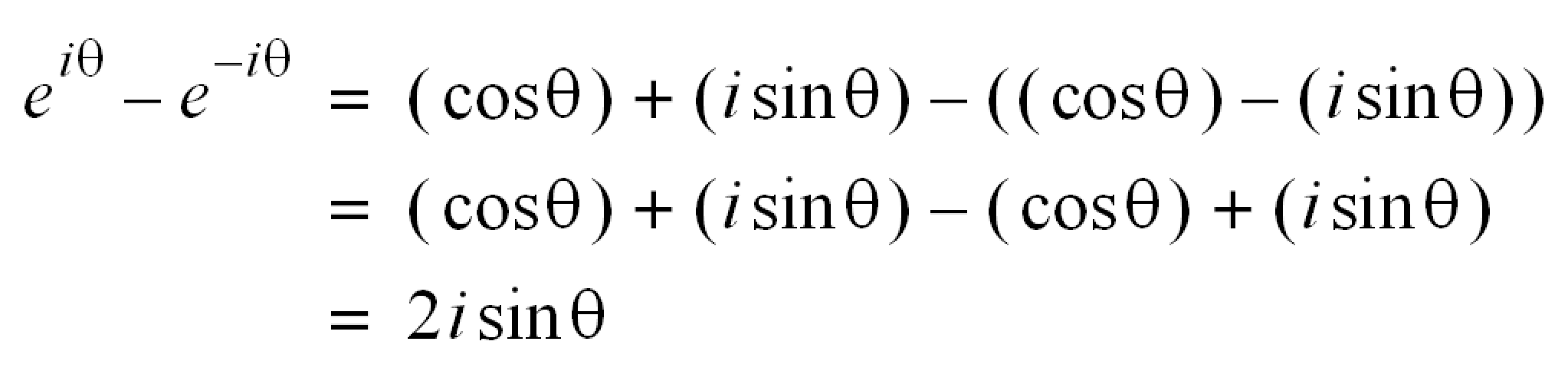p. 83
§ 2.6.12

Incorrect definition of odd function

Second sentence of second full ¶ in §2.6.12, the text reads:

For any x, the positive and negative functions are equal, and sin x = –sin x. Because of this, the sine function is an odd function. In general, a function f is odd if f(x) = –f(x).

The text should read:

For any x, the positive and negative functions are equal, and sin –x = –sin x. Because of this, the sine function is an odd function. In general, a function f is odd if f(–x) = –f(x).

p. 84
fig. 2.25 and table 2.3

Incorrect definition of odd function

The text, figure, and table on this page assert that odd functions are defined as f(x) = –f(x), but again, this is incorrect. The correct definition of an odd function is one in which f(–x) = –f(x). Here are corrected Figure 2.25 and Table 2.3: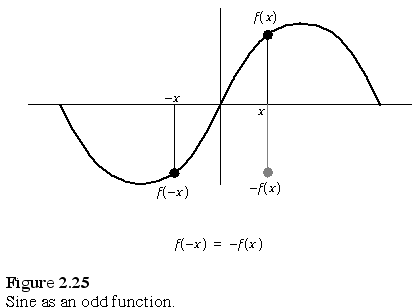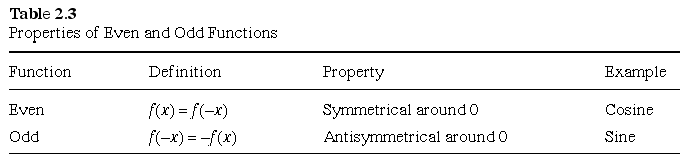p. 89

Incorrect unit of measure

In the final sentence of the page, f2 is given in millihertz (mHz); it should be in megahertz (MHz).

p. 96

Bullet points near bottom of page are incorrect

Bulleted text reads, “Rotate the positive-frequency components of x(t) counterclockwise 90° by multiplying them by i. Rotate the negative-frequency components clockwise 90° by multiplying them by i.”

Text should read: “Rotate the positive-frequency components of x(t) clockwise 90° by multiplying them by i. Rotate the negative-frequency components counterclockwise 90° by multiplying them by i.”

p. 101

Incorrect text in 2nd full paragraph

Text reads: “By investigating conjugate symmetrical phasors, we found that a real cosine is made up of the vector sum of two half-amplitude phasors of opposite frequency. Similarly, a real sine is made up of the vector difference of two imaginary half-amplitude phasors of opposite frequency.”

Change to: “By investigating conjugate symmetrical phasors, we found that a real cosine is made up of the vector sum of two half-amplitude phasors of conjugate symmetry. Similarly, a real sine is made up of the vector difference of two imaginary half-amplitude phasors of conjugate symmetry.”

p. 105
first ¶

Typo

For “clarinetlike”, please substitute “clarinet-like”.

p. 106
Last line of §3.1.6

Wording improvement

For “spectrogram”, please substitute “spectrum”.

p. 109
2nd
bold-italic text

Wording improvement

Text reads, “If the product signal is all positive, then the signals being multiplied must be identical. If the product signal is mixed positive and negative, then the signals being multiplied are not identical.”

Replace with: “The more positive the product signal is, the closer to identical are the source signals. The more mixed positive and negative the product signal is, the less identical are the source signals.”

By “identical”, I mean that the source signals are exactly the same at every point, and do not differ in any property. This means, for example, there is no phase difference between the signals, no difference in DC offset, no amplitude difference, no time-shift difference, no difference in shape. For example, if some x(t) and y(t) signals are not identical, their product will be mixed positive and negative. But if x(t) and y(t) are the same at every point, their product will be all positive (or all 0 if either or both are all zero) at every point.

p. 133
first bullet point in §3.3.8

Clarification

Text reads: “Spectra of negative frequencies and positive frequencies of real signals are mirror images.”

Please substitute: “Magnitudes of the spectra of negative frequencies and positive frequencies of real signals are mirror images.”

p. 134

Incorrect acronym

The inverse discrete Fourier transform is at one point incorrectly given as IFDT; it should be IDFT.

p. 135
matrix figure in §3.4.1

Top and bottom rows are correct. Middle rows show the superscript k+1 in the second column instead of k. Please substitute the corrected matrix figure below.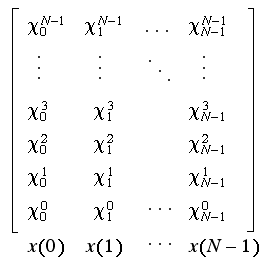p. 136
§ 3.4.3
second ¶

Incorrect assertions

Text reads:

The imaginary part of x(n) will be zero if the imaginary part of X(k) was zero.”

This is incorrect; x(n) will be real if the real part of X(k) is even and the imaginary part is odd relative to frequency 0. Please substitute the following text:

The imaginary part of x(n) will be zero if the real part of X(k) was even and the imaginary part was odd relative to frequency 0.”

p. 136
§ 3.4.4
first ¶

Unclear statement

Text reads:

If the spectrum being processed by the IDFT came from a complex signal, the output of the IDFT may have a significant nonzero imaginary part. But we can still separate the real and imaginary output data in a meaningful way.”

While not really an error, this is unclear, and not really what I wanted to say. What I meant to say is:

Even if the output of the IDFT has a significant nonzero imaginary part, we can still meaningfully separate the real and imaginary output data.”

p. 137
last parenthetical sentence on page

Incorrect assertion

Text reads:

(If the imaginary part of the input spectrum X(k) is zero, computing the imaginary part can be skipped.)”

This is incorrect. The imaginary part being zero is not sufficient. The real part must also be even. Please substitute the following text:

(If the imaginary part of the input spectrum X(k) is zero and the real part is even, then computing the imaginary part can be skipped.)”

p. 140
§ 3.5.1
2
nd

Typo

Text reads:

... increasing the sampling rate R and/or the fundamental analysis frequency N until ...”

Please substitute:

... increasing the sampling rate R and/or the fundamental analysis frequency fN until ...”

p.146
eq. 3.36

Incorrect index for odd function of x

The index for function x in the second summation term should be 2n+1, not 2n–1. Please substitute the following formula for Eq. 3.36.
p. 147 unnumbered equation at top of page

Incorrect index for odd function of x

As with the error on page 146, the index for function x in the odd part should be 2n+1, not 2n–1. Probably a copy/paste error. Please substitute the following formula.
p. 155
first

Incomplete thought

Text reads:

The Hilbert transform of a signal is another signal whose frequency components are all phase shifted by 90º (–π/2 radians).”

Please substitute:

The Hilbert transform of a signal is another signal whose frequency components are all phase shifted by 90º (–π/2 radians for positive frequencies, π/2 for negative frequencies).”

p. 156
Step 3

Typo

Text reads: “Form the elementwise product of X(k) and h(t):”

Should be: “Form the elementwise product of X(k) and h(k):”

p. 163
add sentences to end of 2nd

Improvement

This is not technically an erratum, but some readers have tripped over this point. Please append the following additional explanation to the end of the second paragraph that now ends with the sentence, “This rule even works even when NjNg”:

Recalling that we’ve defined as zero any values that lie outside the range of functions f and g, note that we will get the same result if we set the limit of summation in equation (4.1) to Nf + Ng1 or to any larger value, even to infinity.”

p. 164
§4.8
and
p. 165
§4.9

Convolution sequences are incorrect

The convolution sequences shown in eq. 4.8 and eq. 4.9 are incorrect. Row 1 is OK but rows 2 through 5 should add a leading zero and delete the trailing zero. The summation line has 5+5 elements but should only have 5+5–1 elements. Delete the trailing zero.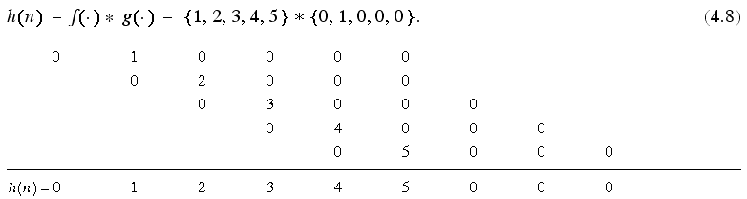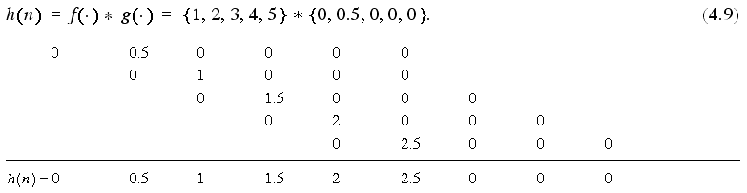p. 168
first equation in §4.4.2

Incorrect font face in equation

First equation in §4.4.2, the font face for F(k) on right side of the equation is incorrect; it should be standard italic font face, as shown corrected here:
It is conventional in mathematics typesetting to use the Corseva font face to indicate the Fourier transform, F .

p. 168
§ 4.4.1

Incorrect term in text

End of first full ¶ in § 4.1.1, text reads:

... indicates that the inverse Fourier transform of spectrum S ( k ) is signal S ( t ).”

Should read:

... indicates that the inverse Fourier transform of spectrum S ( k ) is signal s ( t ).”

p. 171
fig. 4.13

Some values of sampled functions f(n) and g(n) and their product function are incorrect.

The graphical functions in this figure are correct, including the spectral plot.

There are really two problems here.

First, there are transcription errors in the sampled functions f(n) and g(n). More fundamentally, the figure implies that the graphical functions can be reproduced from the sampled functions, which is not true, given how highly truncated the sample values are. Basically, this figure and accompanying text do not provide enough information to adequately demonstrate what is being discussed.

The reader interested in a fuller understanding of the steps shown in this figure and corrected sampled functions are referred to this PDF file. If you prefer, here is the Mathematica notebook. A free Mathematica player for this notebook is available for download here.

p. 172
4th line

Text is in the wrong section

Text reads, “Multiplying in the frequency domain convolves in the time domain.”

True, but this ended up in the wrong section. (A following section talks about multiplying in the frequency domain).

Substitute “Convolving in the frequency domain multiplies signals in the time domain.”

p. 173
text above
eq. 4.15, and
eq. 4.15

Text and equation should both refer to the inverse Fourier transform

Text immediately above Eq. 4.15 and in that equation should both refer to the inverse Fourier transform. Text should read:

Using the notation F 1{ } for the inverse Fourier transform, we can express this operation as:
p. 175

Incorrect function call

In the For loop just under the comment “// Force the spectrum to be conjugate-symmetrical around 0 Hz” the text reads:

RealSet(X[N - k], -Im(X[k]));

but should read

ImagSet(X[N - k], -Im(X[k]));

p. 177
Fig. 4.19

Incorrect word in title

Title reads: “Product of lowpass spectrum and the signal under test.”

Should read: “Product of bandpass spectrum and the spectrum of the signal under test.”

p. 181
first ¶ after
eq. 4.19

Caveat

Please add this text to end of first paragraph after Eq. 4.19:

(For simplicity, this analysis ignores possible scaling asymmetries that may arise, depending precisely upon the particular implementation of the Fourier transform utilized in practice.)”

p. 183
first sentence

Improvement

Text reads: It is a basic premise of physics that periodicity and frequency are ...”

Substitute: “It is a basic premise of physics that period and frequency are ...”

p. 183
eq. 4.21

Typo

Equation begins: “ f ( t ) ”, but it should just be: “ f ”.

p. 191
fig. 4.36 title

Typo

Figure 4.36 title reads: “Sinc squared function and its triangular spectrum.”

Please substitute: “Sinc squared function and its triangular waveform.”

p. 203
last sentence

Incorrect description

Text reads: “The components between the test signals are the result of phase delays introduced by the filter.”

Please substitute: “The components between the test frequencies are the result of artifacts of the analysis method (windowing of the transform with a non-harmonic test signal).”

p. 215 unnumbered display equation just after eq 5.31

Incorrect term in equation

In the unnumbered display equation just after eq. 5.31, three equalities are shown separated by commas. The rightmost term of the middle equality, the term –πT, should be – π / 4T, as shown corrected below.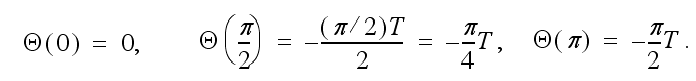p. 215
fig. 5.14

Incorrectly labeled vertical axis in figure

The tick marks on the vertical axis labeled “Phase Shift” should read from top to bottom 0, –π / 4, –π / 2, as shown corrected below.p. 216
eq. 5.32

Incorrect term in equation

The coefficient t in eq. 5.32 should be capitalized, T.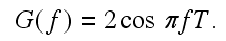p. 217
eq. 5.37

Missing parentheses

Equation 5.37 consists of three lines providing alternate definitions of y(n). The second term of the second equation needs parentheses around the imaginary exponent of e, as shown below. (The third equation line shows parentheses correctly.)

As written: AeiωnT + ϕ

Should be: Aei(ωnT + ϕ)

p. 217 – 248 in some editions

Duplicate pages

It appears that pages 217 to 248 are duplicated in some editions. That's OK, we won't charge you for the extra pages!

p. 218
re. eq. 5.39

Improper range of index

In the ¶ immediately following equation 5.39 the text reads: “... parameter a is a set of M coefficients ...”, however, because the index r ranges from 0 to M in the equation, the text should read “... parameter a is a set of M + 1 coefficients … ”.

p. 227
first line

Incorrect number in inline equation

In the first ¶, the text says “If we set … ω = 2π / 96 …”, but should say “ ω = 2π / 16”.

p. 227
fig. 5.18b

Incorrect figure

Figure 5.18b should show a spiral shrinking counterclockwise.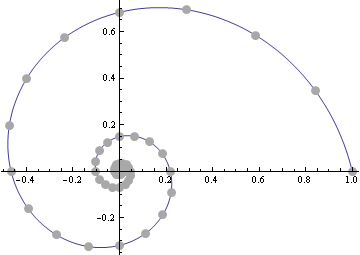p. 227
fig. 5.18c

Incorrect figure

Figure 5.18c should show a spiral expanding counterclockwise.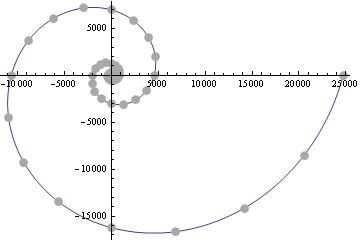p. 227
inline table just before § 5.11.2.

Incorrect descriptions for |z^n| < 1 case
and |z^n| > 1 case

In the two-column inline table just above §5.11.2, the descriptions for the top and bottom relations are incorrect.

The top right-hand entry incorrectly says: “|zn| < 1 Contracting counterclockwise spiral”, but it should say “clockwise:

|z^n| < 1 Contracting clockwise spiral

The bottom right-hand entry incorrectly says: “|zn| > 1 Expanding counterclockwise spiral”, but it should say “clockwise:

|z^n| > 1 Expanding clockwise spiral

p. 228
first ¶
§ 5.11.3

Reference to wrong figures

Mid first paragraph, references to figures 5.18a and 5.18b should instead reference 5.18b and 5.18c, respectively.

Text should read:If we sum the sequence shown in figure 5.18b, ...” “On the other hand, the sum of the sequence in figure 5.18c diverges, ...”

p. 229
text following eq. 5.53

The range of n is misstated

The range of n is misstated in the text following eq. 5.53. The offending sentence reads in part:

For n = 0, 1, 2, …, the sum of all previous terms ...”

but it should read

For n = 1, 2, 3, …, the sum of all previous terms ...”.

p. 229
text in ¶ beginning “If we make sure...”

Textual improvement

Second sentence of the ¶ reads:

It follows that if |r| < 1, then a/(1–r) is closely approximated by Sn ...”

But given that Sn is what we're approximating with a/(1–r), it would be a little clearer if the text said,

It follows that if |r| < 1, then Sn is closely approximated by a/(1–r) ...”

p. 231
¶ immediately before equation 5.59

Terms incorrectly identified in text

The text incorrectly states, “Y(z) is the spectrum corresponding to the impulse response y(n).” Because the impulse response is defined as h(n), therefore its corresponding spectrum is H(z), and Y(z) is actually the spectrum corresponding to the filter output y(n) (that is, the spectrum of the input signal convolved with the impulse response).

p. 234
about 2/3rd of the way down the page

Extra term in inline expression.

Text reads, “By the shift theorem of the Z transform, we can rewrite this term as Y(z) = zmY(z).”

Text should read, “By the shift theorem of the Z transform, we can rewrite the shaded term as zmY(z).”

p. 238
¶ before
eq. 5.71

Incorrect definition of z

The exponent of e should be positive.

The sentence reads: “Now consider what happens when we take equation (5.70), which represents the factored form of H, and set eiωT...”

Please remove the minus sign from the exponent.

Text should read: “Now consider what happens when we take equation (5.70), which represents the factored form of H, and set eiωT...”

p. 239
¶ above
eq. 5.72

Incorrect assertion about e to imaginary powers

Text reads: “and |eanything| = 1”. It should add i to the exponent.

Text should read: “and |ei anything| = 1”

p. 240
3rd line of 1st

Incorrect subscript in formula

The offending formula reads: “|eiωTQ1 |”

It should read: “|eiωTQn |”

p. 241
2nd line

Definition of Θ(ω) is incorrect, misplaced parenthesis

Second line of text reads: “... we can define Θ(ω) = ∠H(e) i ωT.”

Text should read: “... we can define Θ(ω) = ∠H(e i ωT).”

Note the changed location of parentheses and the removed minus sign.

p. 241
bold-italic text

Missing a phrase

Text reads: “... minus the sum of the angles of the vectors to the point e i ωT.”

Should be: “... minus the sum of the angles of the vectors from the poles to the point e i ωT.”

p. 242
last ¶

Incorrect equation setup

Equation 5.78 is correct, but its setup is not. The last ¶ reads,

Setting a = 1 and substituting r = gz, we get” ...

But defining r = gz throws off the derivations shown in eq. 5.78, because this would imply that we'd need parentheses around the term “gz”, which would then change how the signs of the exponents distribute, leading to perdition. Instead, what I should have said is:

Setting a = 1 and substituting r = z, and carrying along our new variable g to scale the position of the pole, we get” …

This allows the derivation shown in eq. 5.78 to work as written.

p. 246
text above
eq. 5.82

Incorrect definition

Text reads: “... we know we can say H(e) = a0 + a1 e.” (note the minus sign in the exponent)

Should be: “... we know we can say H(e) = a0 + a1 e.” (note removed minus sign in the exponent)

p. 246 paperback edition only

Typesetting error in inline equation

Only in the paperback version, there is a typesetting error in the text 6 lines from the bottom.

Text reads in part: “Plotting this, we see that …”, then there is a malformed expression.

The entire sentence with corrected relation should read:

Plotting this, we see that for 0 ≤ ωπ we have the cosine curve shown in figure 5.25.”

p. 248
fig. 5.27 and fig. 4.28

Incorrect z axis values.

The z axis ranges of Figs. 5.27 and 5.28 should be 0 – 2, not 0 – 1, in order to agree with Eq. 5.83 and Figs. 5.25 and 5.26.

p. 248 paperback edition only

Typesetting error in inline equation

In the paperback edition only, below Figure 5.28, some terms of the inline equation for the phase response function Θ(ω) are typeset incorrectly: the sin and cos terms are given in Greek. To wit, “σιν” should be “sin” and “χοσ” should be “cos”.

p. 258
first ¶, last sentence

Improvement

The last sentence of the first full ¶ reads:

So we see that the behavior of the filter when the poles are on the real axis is identical to the one-pole filter.”

Substitute: “So we see that when the poles are on the real axis, this filter behaves similarly to the one-pole filter.”

p. 258
3rd from last ¶

Simplify, remove extraneous concepts

Third full ¶ from bottom, the text reads:

Scaling power by ½ is the same as attenuating power by –3 dB SIL or by –6 dB SPL.”

Please substitute:

Scaling power by ½ is the same as attenuating power by approximately –3 dB SIL.”

p. 262
next to last ¶,
last sentence

Missing a phrase

In the last sentence of the next-to-last ¶, the text reads: “... minus the sum of the angles of the vectors to the same point.”

Should be: “... minus the sum of the angles of the vectors from the poles to the same point.”

(This is another manifestation of the erratum on page 241.)

p. 271
equation at bottom of page

Incorrect term in inline equation

In the bottom inline equation on that page, the incorrect term “ex – 1” is given in the rightmost numerator.

The term should be “ex – 1”.

p. 272
fig. 6.8

Incorrect title text

Text reads: “Plot of e.”

Substitute: “Plot of ex.”

p. 274
just before
eq. 6.15

Typo

Just before eq. 6.15 the text reads: “... then the second derivative of the sine is the negated cosine.”

Should read: “... then the second derivative of the cosine is the negated cosine.”

p. 275
sentence after eq. 6.20

Incomplete formula

In the sentence after eq. 6.20 the text reads: “Thus the acceleration of the air packet is –a2.”

Substitute: “Thus the acceleration of the air packet is –a2 sin aθ.”

p. 278 – 279

Improper value in equations

Setting k = –a in eq. 6.22 was not a good idea. It led to problems in other equations on p. 278 – 279. As the text points out (p. 277), the value of k must be negative “because the slope of the energy curve is negative at all times” for the plucked string example under discussion. But negating k in eq. 6.22 was not the right way to handle it didactically, and doing so also introduced errors.

The simplest fix is to change “–k” back to “k”. Thus, eq. 6.22 should be y(t) = cekt. Then the term “–kin eq. 6.23 becomes just “k, which corrects the error in applying the power rule in that equation. Also please change “–kto “kin eq. 6.24, and also in the first (unlabeled) equation at the top of page 279. Finally, in the first ¶ of §6.2.2, change “y(x) = cektto “y(x) = cekt. Then I think everything works out fine.

p. 281
text following eq. 6.31

Alternative explanation

The text states in part, “Realistically, an educated guess is our only option.” But we can repurpose the text in the immediately preceding section to find the general solution here by way of some complex arithmetic, which (as usual) is more direct than its “simpler” brethren. Start with the Harmonic Equation 6.31: mx''(t) + kx(t) = 0. By 6.29 we can write the solutions for κ1 and κ2 immediately as

κ1 = sqrt ( –4mk) / 2m = i sqrt (k/m) and κ2 = –κ1.

Then eq. 6.32 the Trial Solution is

x(t) = e ^ κ1t and x(t) = e ^ –κ1t.

We know that e^it = cos t + i sin t, so x(t) = cos sqrt (k/m) t + i sin sqrt (k/m) t. This shows more directly where the resonant frequency definition comes from, i.e., sqrt (k/m), and leads directly into the discussion surrounding damped harmonic motion. But if this isn't your cup of tea, the existing text is just fine.

p. 290

Incorrect term in equations

In Section 6.3.3, Driven Harmonic Oscillator, equations 6.53, 6.54, and 6.56 show term cy''( t ), but should show cy'( t ). Correct as follows:

 Eq. 6.53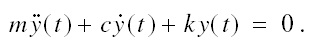Eq. 6.54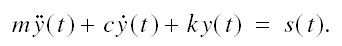Eq. 6.56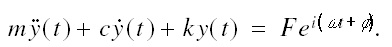p. 295
after eq. 6.63

Add sentence for clarity

Add this (parenthetical) sentence for clarity immediately after Eq. 6.63:

(Though I've dropped the square root from the definition of δ( ω ), the derivative of the positive root is still valid.)”

p. 305
§ 7.1.4

Incorrect terms in equations 7.16 and following

In eq. 7.16, the 2nd function call in numerator: “f (x1 + t1)” should be “f (x1, t1)”, and “lim x → ∞” (limit as x approaches infinity) should be “lim Δx → 0” (limit as Δx approaches zero).

The unnumbered equation directly under eq. 7.16 has a similar problem: “lim t → ∞” should read: “lim Δt → 0”.

p. 311

Incorrect count for matrix

Text reads: “... we end up with an N × M matrix ...”

Substitute: “... we end up with an N + 1 × M + 1 matrix ...”

p. 312
eq. 7.28 and eq. 7.30

Incorrect subscript

The subscript of the rightmost term in the numerator of eq. 7.28 and eq. 7.30 is incorrect.

Eq. 7.28 should be: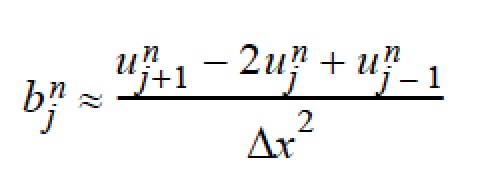and Eq. 7.30 should be: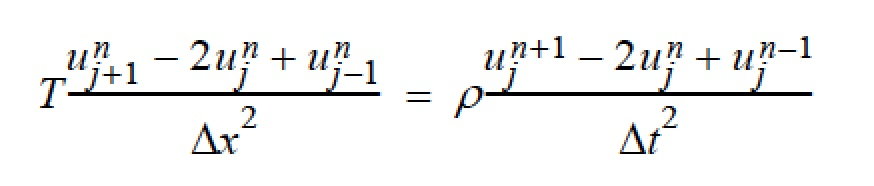p. 314
mid-page

Incorrect term in equations

From the ¶ beginning “To evaluate the impact ...” over to and including the first equation on the next page, the ordinary derivative equations should all be of y with respect to t instead of u with respect to t because we are differentiating the function y = A sin ωt. For example, the first ordinary differential equation (shown mid-page) would be: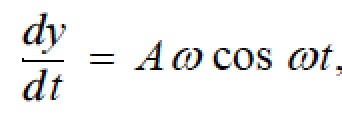and the rest of the ordinary differential equations through the end of that section would likewise be with respect to y. The partial differential equation 7.36 would remain with respect to u.

p. 348
after eq. 8.34

Incorrect sentence

Just after eq. 8.34, the text says, “This is the distance a wave travels over a unit length D.” Just delete that sentence. The sentence before eq. 8.34 says it better: “The velocity of a wave over some distance D may be found with ...”.

p. 353
second from last line

Incorrect word

Text reads: “Since the amount of power available to the loudspeaker from the battery...”

Substitute: ““Since the amount of power available to the loudspeaker from the oscillator...”

p. 356
fig. 8.26

Fontosis in figure

The music fonts in the two staves at the top right of the figure are incorrect. The correct figure is shown here.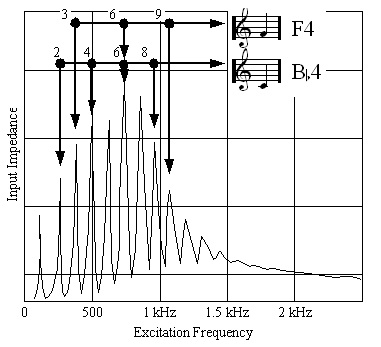p. 368
eq. 9.2

Improvement

The range for t on the lower line of stacked eqations 9.2 is given as ta, but it should be given as ata+d.

p. 369
fig. 9.7

The x axis maximum is incorrect

In Figure 9.7, the point at which the waveform goes to zero should be 0.7, not 1. Here is just the corrected right-hand part of the figure.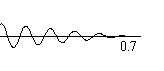p. 374
inline table

Inline table mid-page incorrectly gives frequency as DC

Mid-page, for k = 0 the inline table incorrectly gives the frequency as DC. The correct frequency for k = 0 is 1.

The text should read (only first 4 columns shown here):
p. 375
inline table

Inline table at bottom of page incorrectly gives frequency as DC

Bottom of page, for k = 0 the inline table incorrectly gives the frequency as DC. The correct frequency for k = 0 is 1.

Text should read (only first 4 columns shown here):
p. 376
eq. 9.12

Improvement

The equation shown for the non-band-limited triangular wave, while correct, does not have the same phase as Eq. 9.11, the band-limited triangular wave. The following formula provides the same phase as Eq. 9.11:

f ( t ) = 2 | tπ | / π – 1,

but it is limited in range to 0 ≤ t2π. To remove these limitations, if desired, we can replace Eq. 9.12 with the following:

f ( t ) = 2 | 2 t / ( 2 π ) 1 | – 1

where ...⎦ is the floor function.

p. 376
eq. 9.15

Typo

In eq. 9.15, t(t) should instead be f(t).

p. 380

Incorrect formula for linear interpolation

There are two errors on this page. First, near the end of the last full ¶ the text reads:

In this example, the interpolated result would be y = 0.7 y14 + (1 – 0.7) y15 = 4.11”,

The text should read, “In this example, the interpolated result would be y = 0.7 y15 + (1 – 0.7) y14 = 4.11”.

So the subscripts 14 and 15 should be swapped. Second, just below this the text reads:

Step 2: y = σys⎦ + 1 – σys+1⎦,

but text should read:

Step 2: y = σys+1⎦ + (1 – σ)ys⎦.

p. 381
mid-page

Questionable assertion

Text says, “Spectrally, linear interpolation is equivalent to a second-order lowpass filter with a triangular impulse response.”

Substitute: “Spectrally, linear interpolation is similar to a second-order lowpass filter with a triangular impulse response.”

p. 383
eq. 9.24

Missing subscript on equation term

In Step 1 of eq. 9.24, term A should be An.

p. 385
fig. 9.20

Printing bug

The first cycle of the function in fig. 9.20 has an unexpected straight segment followed by a corner. This looks to have been an error in the software I used to create the figure.

p. 390
fig. 9.29a

Bounding arrows are too small to see

The negative-frequency spectrum of Fig. 9.29a shows two tiny bumps to the left and right of the line for –1000 Hz. These bumps are actually the heads of a double-headed arrow that is showing the extent of the modulating frequency fm , but they came out too small to see on the printed page. The figure fragment shown here corrects this appearance by replacing the double-headed arrows with two single-headed arrows bracketing the modulating frequency fm.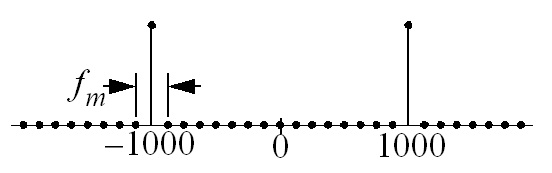p. 416
eq. 9.47

Extraneous term in matrix

Remove the term b0 from the middle bracketed term. Equation 9.47 should look like this: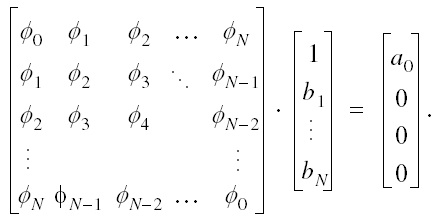p. 421
§ 9.5.3
eq. 9.49

Incorrect term in equation

In the middle stacked equation, the term “1 – cos ( β t )”, should be “1 – cos ( β n )”.

p. 422
fig. 9.52

Incorrect subscripts in figure

Subscripts on the parameters for the 2nd patch bank should be subscripted “2” in order to show a progression to N in the bottom row of the patch. So β1 α1 ω1 and ϕ1 should be β2 α2 ω2 and ϕ2.

p. 423

Printer's error in paperback edition

Only in the paperback edition of V2: the last two lines of p. 422 are repeated at the top of p. 423. This printer’s error was introduced by the MIT Press when they put out the paperback edition; it does not appear in the hardbound edition.

p. 441
fig. 9.71

Incorrect subscript in equation in figure

The equation for R0,1 is missing term Z0. The equation should be written as (Z1 – Z0) / (Z1 + Z0), as shown here.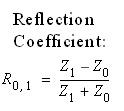p. 441
§ 9.7.3
2nd

Off-by-one error

The 2nd paragraph of that section, 3rd sentence reads: “If we sample these traveling wave components at N equidistant points ...”

This should read: “If we sample these traveling wave components at N+1 equidistant points ...”

The 3rd paragraph of that section:

Using the terminology of stringed instruments … and the other end the nut termination at position x = N1”

This should read:

Using the terminology of stringed instruments … and the other end the nut termination at position x = N since it's length is N.”

p. 462
fig. 10.10

The upper “board” is time-reversed

The figure doesn't show x(–1)h(1) as described. Flipping the top row about h(0) (time-reversing) fixes it, as shown here: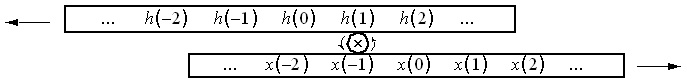p. 463

Misspellings

In the 4th line below eq. 10.7, “analized” should be “analyzed”. In that same line, “monotically” should be “monotonically”.

p. 465
fig. 10.13.a

Incorrect spectrum in figure

Spectrum should be shifted by k/N so it's modulated to 0 Hz. Here's the corrected figure.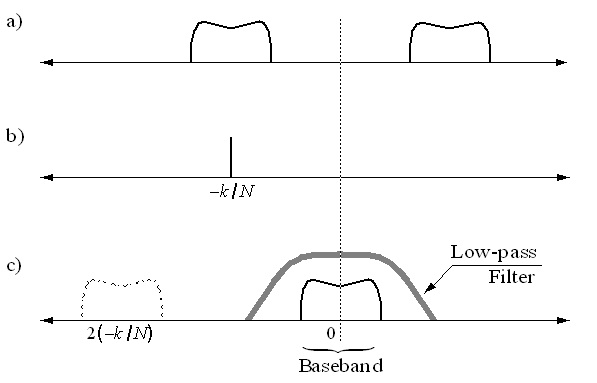p. 465
last two equations, and
p. 466
first equation

Incorrect derivations

The last two equations on page 465 and first equation on 466 should contain h(r), not h(–r)
based on the substitution r = nr.

This makes things a little easier on page 466. We can delete the 2nd paragraph, beginning “The negative index for h(–r)...” through and including the following equation that ends “if and only if h(r) = h(–r).”

Also, in equation 10.11, we can delete the text “if and only if h(r) = h(–r).”

p. 479
eq. 10.18

Improper substitution

Equation 10.18 is supposed to be the same as equation 10.4 with the substitution n = sR. However, I got carried away and also substituted r = sR in the superscript of e. The superscript shown, – I 2 π k ( s R ) / N, is incorrect, and should be – I 2 π k r / N. The corrected formula is shown here:
p. 521 unlabeled display equation above eq. A.20

Missing parentheses

Parentheses are missing after the coefficient i. The equation should be: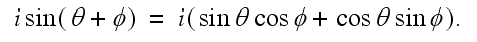# Errata Rewards for Musimathics

I have adopted Donald Knuth's reward system to encourage readers to report errors in Musimathics. The first finder of any error receives $2.56 (one hexadollar). Significant suggestions are also worth$0.32 each.
Knuth notes that, “If you are really a careful reader, you may be able to recoup more than the cost of the books this way.”

Please send your comments by electronic mail to Errata <~< at >~> Musimathics.com.

You can also communicate with me by snail mail:

Gareth Loy
POB 151185
San Rafael, CA 94915.

I will attempt to reply to all such messages within six weeks of receipt.

Please do not send email to the above address except to report errors in Musimathics.  If you report an error via email, please do not include attachments of any kind, and use plain ASCII  text.  For representing mathematical equations in plain text, I suggest http://mathforum.org/dr.math/faq/faq.typing.math.html or http://www.karlscalculus.org/email.html.  The character set should be Western.

I'm very sorry, but I am not sufficiently fluent in any language besides English to discuss anything more complicated than travel directions. Though I can usually make out French and Spanish if I work at it, it will probably be best for all concerned if you communicate with me in English. Sorry.

Regards,
Gareth Loy